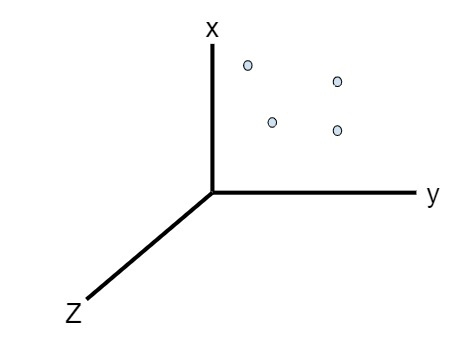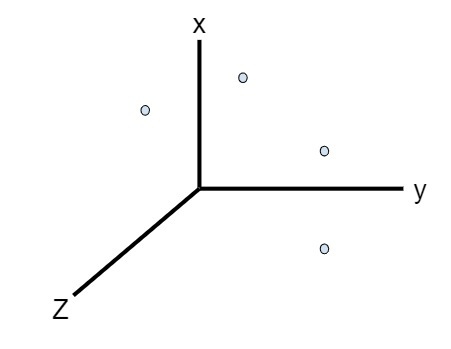# C++ Program to check whether points in a 3-D plane are Coplanar

Given with the points (x1, y1, z1), (x2, y2, z2), (x3, y3, z3) and (x4, y4, z4) and the program must check whether the given points are coplanar or not. Points are said to be coplanar is they lie under same plane and if they are under different-2 planes than the points are not coplanar.

Given below is an image containing four points and all are under same plane which is xy plane that means points are coplanarGiven below is an image containing four points and all are under different planes which shows points are not coplanar## Example

Input-: x1 = 2, y1 = 3, z1 = 1, x2 = 1, y2 = 9, z2 = 3, x3 = 3, y3 = 1, z3 = 5, x4 = 23, y4 = 21, z4 = 9
Output-: they are not coplanar
Input-: x1 = 3, y1 = 2, z1 = -5, x2 = -1, y2 = 4, z2 = -3, x3 = -3, y3 = 8, z3 = -5, x4 = -3, y4 = 2, z4 = 1
Output-: they are coplanar

Approach used in the below program is as follows

• Input the points in the variables (x1, y1, z1), (x2, y2, z2), (x3, y3, z3) and (x4, y4, z4)
• Find the equations of the plane and check whether they are satisfying the conditions or not
• Display whether the points are coplanar or not

## ALGORITHM

START
Step 1-> declare function to check whether points in 3-D are coplanar or not
void check_coplanar(int x1,int y1,int z1,int x2,int y2,int z2, int x3, int y3, int z3, int x, int y, int z)
declare variable as int a1 = x2 - x1
declare variable as int b1 = y2 - y1
declare variable as int c1 = z2 - z1
declare variable as int a2 = x3 - x1
declare variable as int b2 = y3 - y1
declare variable as int c2 = z3 - z1
declare variable as int a = b1 * c2 - b2 * c1
declare variable as int b = a2 * c1 - a1 * c2
declare variable as int c = a1 * b2 - b1 * a2
declare variable as int d = (- a * x1 - b * y1 - c * z1)
check
IF(a * x + b * y + c * z + d = 0)
print coplanar
End
Else
print not coplanar
End
Step 2-> In main()
declare and set variable int x1 = 2 , y1 = 3, z1 = 1, x2 = 1, y2 = 9, z2 = 3, x3 = 3, y3 = 1, z3 = 5, x4 = 23, y4 = 21, z4 = 9
call check_coplanar(x1, y1, z1, x2, y2, z2, x3,y3, z3, x4, y4, z4)
STOP

## Example

#include<bits/stdc++.h>
using namespace std ;
//calculate points in a plane are coplanar or not
void check_coplanar(int x1,int y1,int z1,int x2,int y2,int z2, int x3, int y3, int z3, int x, int y, int z) {
int a1 = x2 - x1 ;
int b1 = y2 - y1 ;
int c1 = z2 - z1 ;
int a2 = x3 - x1 ;
int b2 = y3 - y1 ;
int c2 = z3 - z1 ;
int a = b1 * c2 - b2 * c1 ;
int b = a2 * c1 - a1 * c2 ;
int c = a1 * b2 - b1 * a2 ;
int d = (- a * x1 - b * y1 - c * z1) ;
if(a * x + b * y + c * z + d == 0)
cout << "they are coplanar" << endl;
else
cout << "they are not coplanar" << endl;
}
int main() {
int x1 = 2;
int y1 = 3 ;
int z1 = 1 ;
int x2 = 1 ;
int y2 = 9 ;
int z2 = 3 ;
int x3 = 3 ;
int y3 = 1 ;
int z3 = 5 ;
int x4 = 23 ;
int y4 = 21 ;
int z4 = 9 ;
check_coplanar(x1, y1, z1, x2, y2, z2, x3,y3, z3, x4, y4, z4) ;
return 0;
}

## Output

If run the above code it will generate the following output -

they are not coplanar